# fir analysis

## Introduction

Dave Fournier suggests that “NB1” (i.e., a negative binomial model parameterized so that the variance is proportional to the mean, rather than the more traditional “NB2” with $$V= \mu(1+\mu/k)$$) is a better fit to the fir data set from Dodd and Silvertown 1999 (reproduced in Bolker 2008 Ecological Models and Data). He points out that NB2 is overwhelmingly more popular in the R community (and to be fair the statistics literature in general, although I see that SAS's PROC COUNTREG allows fitting of the NB1 model …), probably because NB2 with a known overdispersion parameter fits into the exponential family that can be handled by the generalized linear model framework.

## Preliminaries

Load various useful packages/preliminary data manipulation:

library(emdbook)
firdat <- na.omit(FirDBHFec[, c("TOTCONES", "DBH", "WAVE_NON")])
firdat <- transform(firdat, TOTCONES = round(TOTCONES))
library(ggplot2)
theme_set(theme_bw())
library(bbmle)
library(reshape2)


A basic plot (although in this example we will ignore the distinction between “wave” and “non-wave” populations, at least to begin with)

qplot(DBH, TOTCONES, colour = WAVE_NON, shape = WAVE_NON, data = firdat)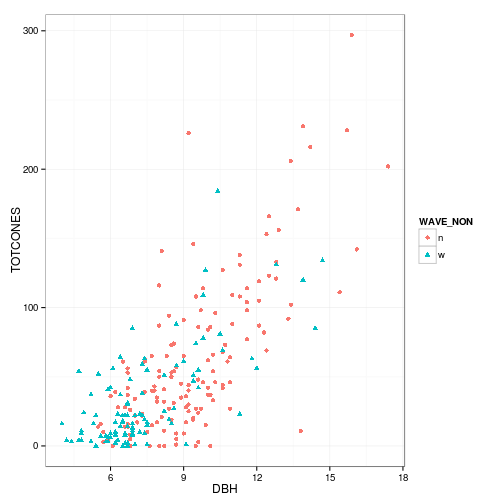## NB2

We'll start by fitting the more traditional NB2 model.

Fitting with ADMB, using the TPL file found in Mollie Brooks' example on the ADMB project web site:

compile_admb("fir0")
write_dat("fir0", with(firdat, list(nobs = nrow(firdat), totcones = TOTCONES,
dbh = DBH)))
write_pin("fir0", list(a = 1, b = 1, k = 1))  ## fails with k=5 start


FIXME: check order vs. TPL in write_dat !!

Now try it in R, both with mle2 and with glm.nb from the MASS package:

r1 <- mle2(TOTCONES ~ dnbinom(mu = a * DBH^b, size = k), start = list(a = 1,
b = 1, k = 1), data = firdat, method = "Nelder-Mead")
r2 <- MASS::glm.nb(TOTCONES ~ log(DBH), data = firdat)


It takes slightly more work to extract the glm.nb coefficients in the same format as the other models:

r2coef2 <- with(as.list(coef(r2)), c(a = exp((Intercept)), b = log(DBH),
k = r2$theta))  One of the nice things about glm.nb (despite its limitations) is that it makes diagnostic plots easy: par(mfrow = c(2, 2), mar = c(4, 4, 1.5, 1), las = 1, bty = "l", mgp = c(2, 1, 0)) colvec <- adjustcolor(c("blue", "purple"), alpha.f = 0.6) plot(r2, lwd = 2, col = colvec[firdat$WAVE_NON])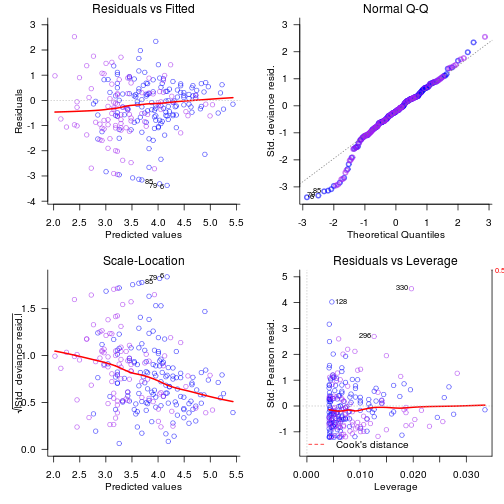The most obvious problem is in the scale-location plot (lower left), where the decreasing line shows that our model variance increases faster than it should with the mean (so that the scaled residual variance decreases with the mean). This is a strong suggestion that the linear mean-variance relationship of NB1 might be more appropriate than the quadratic relationship of NB2 …

The Q-Q plot also has a wonky little wiggle in it. I'm not sure where this comes from – it may be related to the variance scaling problem. I worried that it might be related to the population grouping we were ignoring, so I tried looking at the Q-Q plot split by population (code taking from the examples in ?qqmath):

firdat$resid <- residuals(r2) lattice::qqmath(~resid | WAVE_NON, data = firdat, prepanel = prepanel.qqmathline, panel = function(x, ...) { panel.qqmathline(x, ...) panel.qqmath(x, ...) })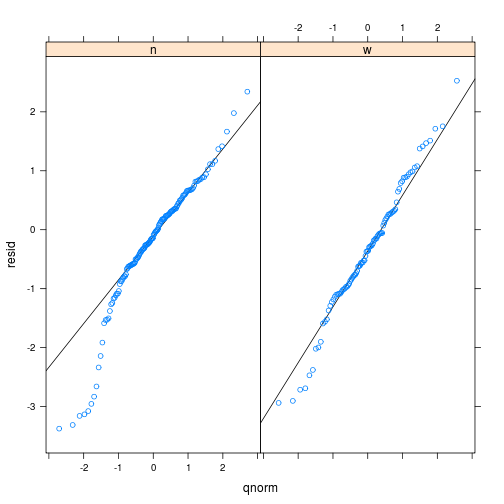## NB1 Let's try NB1. We want $$V=\tau \mu$$ rather than $$V=\mu(1+\mu/k)$$, hence to convert between types we should take $$(1+\mu/k)=\tau$$, or $$k=\mu/(\tau-1)$$. To do this most conveniently in mle2 we define a new density function for NB1: dnbinom1 <- function(x, mu, tau, log = FALSE) { k <- mu/(tau - 1) dnbinom(x, mu = mu, size = k, log = log) } r3 <- mle2(TOTCONES ~ dnbinom1(mu = a * DBH^b, tau), start = list(a = 1, b = 1, tau = 5), data = firdat, method = "Nelder-Mead")  DF actually used a robust NB1 model, where we assume a mixture of two different NB1 models with different $$\tau$$ values. compile_admb("firxr") write_dat("firxr", with(firdat, list(nobs = nrow(firdat), totcones = TOTCONES, dbh = DBH))) write_pin("firxr", list(a = 0.3, b = 2, tau = 27, p = 0.05, w = 9)) run_admb("firxr") admb2 <- read_admb("firxr")  FIXME: make ADMB log-likelihoods get proper dfs coef(r3)  ## a b tau ## 0.3646 2.2438 27.5132  AICtab(r1, r3)  ## dAIC df ## r3 0.0 3 ## r1 15.2 3  firdat$predict2 <- with(as.list(coef(r3)), a * firdat$DBH^b) firdat <- within(firdat, { predict2 <- with(as.list(coef(r3)), a * DBH^b) resid2_unsc <- predict2 - TOTCONES p2_var <- coef(r3)["tau"] * predict2 resid2 <- resid2_unsc/sqrt(p2_var) }) qplot(predict2, sqrt(abs(resid2)), data = firdat) + geom_smooth(colour = "red", se = FALSE, method = "loess")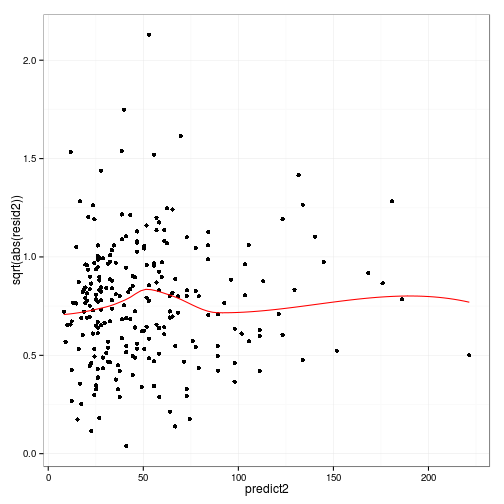qplot(sample = resid2, data = firdat)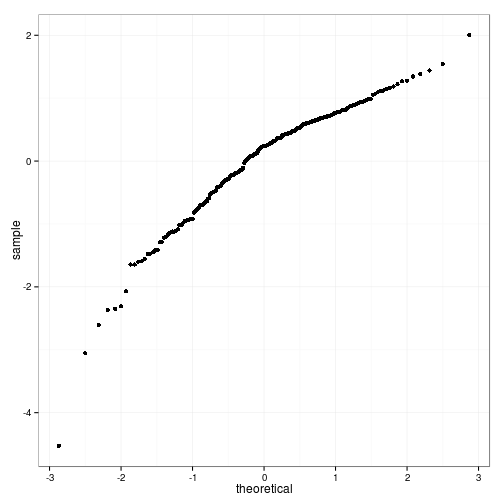Q-Q plot is still not perfect, but better … ## Scaling plots Look at residuals from an nls fit (assuming constant variance), see how n1 <- nls(TOTCONES ~ a * DBH^b, data = firdat, list(a = 1, b = 1)) ndat <- data.frame(v = residuals(n1)^2, m = fitted(n1)) f1 <- lm(v ~ m - 1, data = ndat) f2 <- lm(v ~ I(m^2) + offset(m) - 1, data = ndat) pp <- data.frame(m = seq(min(ndat$m), max(ndat\$m), length = 101))
pp <- data.frame(pp, NB1 = predict(f1, newdata = pp), NB2 = predict(f2, newdata = pp))
mpp <- melt(pp, id.var = 1, value.name = "v")
g1 <- qplot(m, v, data = ndat) + geom_line(data = mpp, aes(colour = variable))
g1 + geom_smooth(method = "loess", colour = "purple") + coord_cartesian(ylim = c(0,
16000))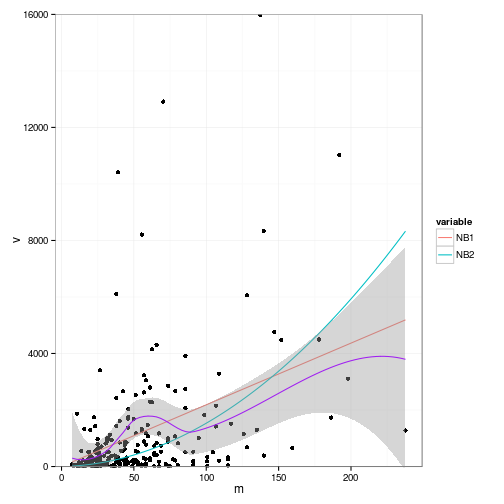(Axis limits exclude one point at approx. m=50, v=30000)

On a log-log plot, it looks as though NB2 fits better …

g1 + scale_y_log10() + scale_x_log10()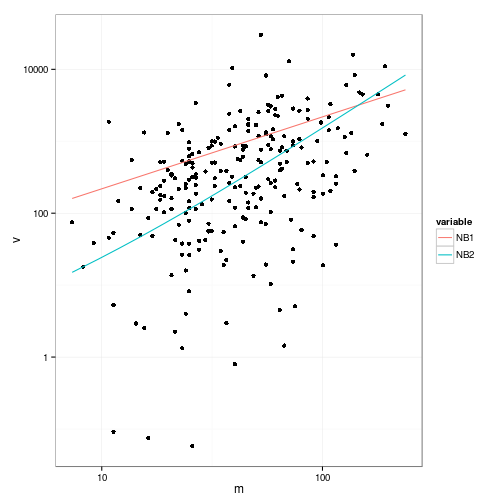## Predictions

How much do the coefficient estimates of all three (NB2, NB1, robust NB1) differ … ?

library(coefplot2)

## Loading required package: coda

modList <- list(NB2.R = r1, NB2.ADMB = admb1, NB1.R = r3, NB1rob.ADMB = admb2)
cList <- lapply(lapply(modList, coeftab), function(x) x[c("a", "b"), ])
colvec <- c(1, 2, 4, 5)
coefplot2(cList, intercept = TRUE, col = colvec, legend = TRUE)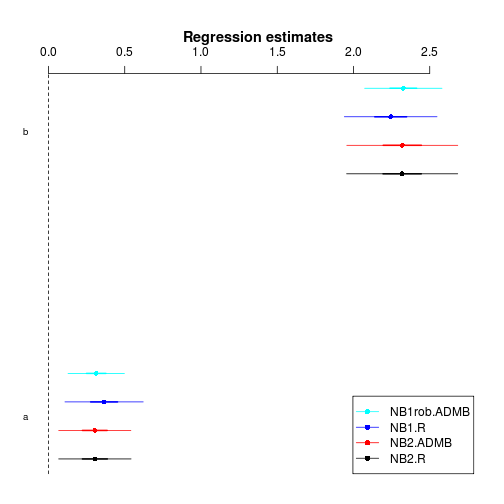Not much …